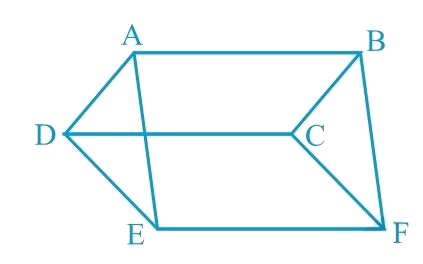# Ex.9.4 Q3 Areas of Parallelograms and Triangles Solution - NCERT Maths Class 9

Go back to  'Ex.9.4'

## Question

In the following figure, $$ABCD$$, $$DCFE$$ and $$ABFE$$ are parallelograms. Show that ar ($$\Delta \rm{ADE}$$) $$=$$ ar ($$\Delta \rm{BCF}$$).

Video Solution
Areas Of Parallelograms And Triangles
Ex 9.4 | Question 3

## Text Solution

What is known?

$$ABCD$$,$$DCFE$$ and $$ABFE$$ are parallelograms.

What is unknown?

How we can show that $$\text{ar }\left( \Delta \text{ADE} \right)\text{ = ar }\left( \Delta {BCF} \right)\text{.}$$

Reasoning:

We can see that sides of triangles $$ADE$$ and $$BCF$$ are also the opposite sides of the given parallelogram. Now we can show both the triangles congruent by SSS congruency. We know that congruent triangles have equal areas.Steps:

It is given that $$ABCD$$ is a parallelogram. We know that opposite sides of a parallelogram are equal.

$$\therefore \rm A D=B C \ldots(1)$$

Similarly, for parallelograms $$DCFE$$ and $$ABFE$$, it can be proved that

$$DE=CF$$.$$...(2)$$

And, $$EA = FB$$$$... (3)$$

In $$\Delta {ADE}$$ and $$\Delta {BCF}$$,

$$AD = BC$$ [Using equation ($$1$$)]

$$DE = CF$$ [Using equation ($$2$$)]

$$EA = FB$$ [Using equation ($$3$$)]

$$\therefore \Delta ADE \cong \Delta BCF\!\! \begin{pmatrix} \!\text { SSS} \!\\ \!\text{congruence } \!\\ \!\text{rule } \! \end{pmatrix}$$

The SSS Rule States that : If three sides of one triangle are equal to the three sides of another triangle, then the two triangles are congruent.

$\therefore \text { Area }(\Delta {ADE})=\text { Area }(\Delta {BCF})$

Video Solution
Areas Of Parallelograms And Triangles
Ex 9.4 | Question 3

Learn from the best math teachers and top your exams

• Live one on one classroom and doubt clearing
• Practice worksheets in and after class for conceptual clarity
• Personalized curriculum to keep up with school